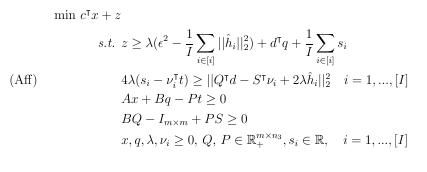# SOCP reformulation

I have the following SOCPwith code in matlab
function [optval,rtime]=TwoStageAffine(n,m,N,c,d,epsilon,A,B,h,S,t)
o=length(t);
M= (1/N)*sum(sum(h.^2,2));
tic
cvx_begin quiet
variable x(n) nonnegative;
variable Q(n,m);
variable q(n) nonnegative;
variable z(1);
variable s(N);
variable l(1) nonnegative;
variable v(o,N) nonnegative;
variable P(m,o) nonnegative;
variable W(2,2,N);

``````minimize(c.'*x+z);
subject to
z>= l*(epsilon^2-M)+d.'*q+(1/N)*sum(s);
A*x+B*q-P*t>=0;
B*Q-eye(m)+P*S>=0;
for i=1:N
expression WW1(1);
expression WW2(1);
WW1==4*l*(s(i)-v(:,i).'*t);
WW2==norm(Q.'*d-S.'*v(:,i)+2*l*h(:,i));
W(:,:,i)==[WW1,WW2;WW2,1];
W(:,:,i)==semidefinite(2,2);
end
``````

cvx_end
I’m getting the error: “Disciplined convex programming error: Invalid quadratic form(s): not a square.”
I know the issue is on the norm constraint and the fact that \lamba (variable) is multiplied by both s_i and v_i, both variables. I have it running using gurobi in pyhton but for simulations reasons it wil be more convenient to have it in matlab. Yet I still don’t know who to plug it in to CVX properly.